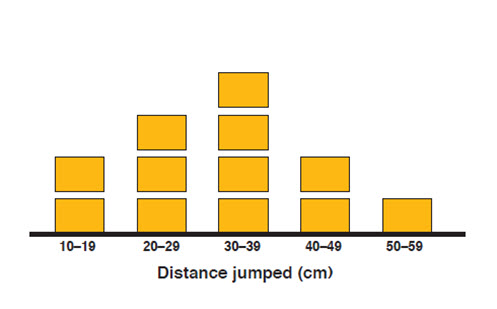Go to website

# reSolve: Measurement: Jump!

In this sequence of two lessons, students investigate how far they can jump and explore the jumping distance of a range of animals. Students first estimate the distance they can jump, then undertake an investigation by jumping using a range of techniques. Class data is recorded and displayed and students compare their jumping distances. They then learn about the jumping distance of several animals, and use the concept of scale to estimate the lengths of jumps they would make if they were those animals. Each lesson is outlined in detail including curriculum links, vocabulary, materials needed, sample answers, discussion points and student resources. This sequence is part of the reSolve: Mathematics by Inquiry program.

Year level(s) Year 3
Audience Teacher
Purpose Teaching resource
Teaching strategies and pedagogical approaches Mathematics investigation
Keywords data representation, multiplication, collecting data, length

## Curriculum alignment

Curriculum connections Numeracy
Strand and focus Number, Measurement, Statistics, Build understanding, Apply understanding
Topics Multiplication and division, Using units of measurement, Data representation and interpretation
AC: Mathematics (V9.0) content descriptions
AC9M3N04
Multiply and divide one- and two-digit numbers, representing problems using number sentences, diagrams and arrays, and using a variety of calculation strategies

AC9M3M01
Identify which metric units are used to measure everyday items; use measurements of familiar items and known units to make estimates

AC9M3ST01
Acquire data for categorical and discrete numerical variables to address a question of interest or purpose by observing, collecting and accessing data sets; record the data using appropriate methods including frequency tables and spreadsheets

Numeracy progression Multiplicative strategies (P5)
Understanding units of measurement (P6)
Interpreting and representing data (P4)